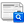Journal of the European Optical Society - Rapid publications, Vol 8 (2013)

MAP entropy estimation: applications in robust image filtering

J. I. de la Rosa, J. Villa-Hernandez, E. de la Rosa M., E. Gonzalez-Ramirez, O. Gutierrez, N. Escalante, R. Ivanov, G. Fleury

Abstract

We introduce a new approach for image filtering in a Bayesian framework. In this case the probability density function (pdf) of thelikelihood function is approximated using the concept of non-parametric or kernel estimation. The method is based on the generalizedGaussian Markov random fields (GGMRF), a class of Markov random fields which are used as prior information into the Bayesian rule, whichprincipal objective is to eliminate those effects caused by the excessive smoothness on the reconstruction process of images which arerich in contours or edges. Accordingly to the hypothesis made for the present work, it is assumed a limited knowledge of the noise pdf,so the idea is to use a non-parametric estimator to estimate such a pdf and then apply the entropy to construct the cost function for thelikelihood term. The previous idea leads to the construction of Maximum a posteriori (MAP) robust estimators, since the real systems arealways exposed to continuous perturbations of unknown nature. Some promising results of three new MAP entropy estimators (MAPEE) forimage filtering are presented, together with some concluding remarks.

Full Text: PDF

Citation DetailsCite this article

References

N. Bertaux, Y. Frauel, P. Réfrégier, and B. Javidi, ”Speckle removal using a maximum-likelihood technique with isoline gray-level regularization,” J. Opt. Soc. Am. A 21(12), 2283–2291 (2004).

J. E. Besag, ”Spatial interaction and the statistical analysis of lattice systems,” J. Royal Stat. Soc. Ser. B B-36, 192–236 (1974).

J. E. Besag, ”On the statistical analysis of dirty pictures”, J. Royal Stat. Soc. Ser. B B-48, 259–302 (1986).

C. Bouman, and K. Sauer, ”A generalizaed Gaussian image model for edge-preserving MAP estimation,” IEEE T. Nucl. Sci. 99(4), 1144– 1152 (1992).

K. Champagnat, and J. Idier, ”A conection between half-quadratic criteria and EM algorithms,” IEEE Signal Proc. Let. 11(9), 709–712 (2004).

S. Geman, and C. Geman, ”Stochastic relaxation, Gibbs distribution, and the Bayesian restoration of images,” IEEE T. Pattern Anal. 6, 721–741 (1984).

K. Sauer, and C. Bouman, ”Bayesian estimation of transmission tomograms using segmentation based optimization,” IEEE T. Image Process. 2(3), 296–310 (1993).

J. I. De la Rosa, and G. Fleury, ”On the kernel selection for minimum-entropy estimation,” in Proceedings of the IEEE Instrumentation and Measurement Technology Conference, 1205–12010 (IEEE, Anchorage, 2002).

J. I. De la Rosa, G. Fleury, and M.-E. Davoust, ”Minimum-entropy, pdf approximation and kernel selection for measurement estimation,” IEEE T. Instrum. Meas. 52(4), 1009–1020 (2003).

J. I. De la Rosa, ”Convergence of minimum-entropy robust estimators: Applications in DSP and instrumentation,” in Proceedings of the XIV International Conference on Electronics, Communications, and Computers - CONIELECOMP’04, 98–103 (IEEE, Veracruz, 2004).

P. Milanfar ”A Tour of Modern Image Filtering,” IEEE Signal Proc. Mag. 30(1), 106–128, Jan. (2013).

H. Takeda, S. Farsiu, and P. Milanfar, ”Kernel regression for image processing and reconstruction,” IEEE T. Image Process. 16(2), 349– 366, Feb. (2007).

Ö. Egecioglu, and A. Srinivasan, ”Efficent non-parametric density estimation on the sphere with applications in fluid mechanics,” SIAM J. Sci. Comput. 22(1), 152–176 (2000).

G. P. Terrell, ”The maximal smoothing principle in density estimation,” J. Am. Stat. Assoc. 85, 470–477 (1990).

G. P. Terrell, and D. W. Scott, ”Oversmoothed nonparametric density estimation,” J. Am. Stat. Assoc. 80, 209–214 (1985).

L. Pronzato, and E. Thierry, ”A minimum-entropy estimator for regression problems with unknown distribution of observation errors,” in textslMaxEnt 2000, Eds. A. Mohammad-Djafari, 169–180 (American Institute of Physics, College Park, 2000).

L. Pronzato, and E. Thierry, ”Entropy minimization of parameter estimator with unknown distribution of observation errors,” in Proceedings of the IEEE International Conference in Acoustics, Speech and Signal Processing, 3993–3996 (IEEE, Salt Lake City, 2001).

E. Masry, ”Probability density estimation from sampled data,” IEEE T. Inform. Theory IT-29(5), 697–709 (1983).

L. Devroye, ”A note on the usefulness of superkernels in density estimation,” Ann. Stat. 20, 2037–2056 (1992).

L. Devroye, ”The double kernel method in density estimation,” Ann. I. H. Poincare 25, 533–580 (1989).

L. Devroye, and A. Krzyza˙ k, ”On the Hilbert kernel density estimate,” Stat. Probabil. Lett. 44, 299–308 (1999).

L. Devroye, ”Universal smoothing factor selection in density estimation: Theory and practice,” Test 6, 223–320 (1997).

A. Berlinet, and L. Devroye, ”A comparison of kernel density estimates,” Publications de l’Intitut de Statistique de l’Université de Paris 38(3), 3–59 (1994).

C. M. Loader, ”Bandwidth selection: classical or plug-in?,” Ann. Stat. 27(3), 415–438 (1999).

E. Wolsztynski, E. Thierry, and L. Pronzato, ”Minimum entropy estimation in semi parametric models,” Signal Process. 85, 937–949 (2005).